# 如何在TensorFlow中用深度学习修复图像？

## 目录

• 介绍

• 第一步：把图像解析成概率分布中的样本点

• 第二步：快速生成伪造图像

• 第三步：找到修复图像的最佳伪造图片

• 结论

1. 我们首先要把图像解析成概率分布中的样本点

2. 这种解析让我们学习如何产生伪图像

3. 接着我们就能找到补全修复所需的最佳伪图像## 第一步：把图像解析成概率分布中的样本点

1. 环境信息：你可以通过周围像素点的信息推断出缺失的像素点是什么。

2. 知觉信息：你会填补一些你认为是「正常」的内容，比如你在现实生活中或其他图像中可能看到的东西。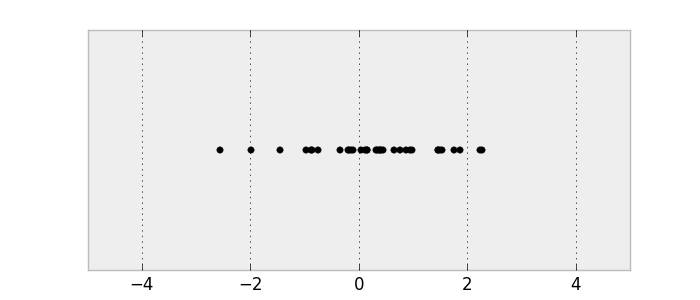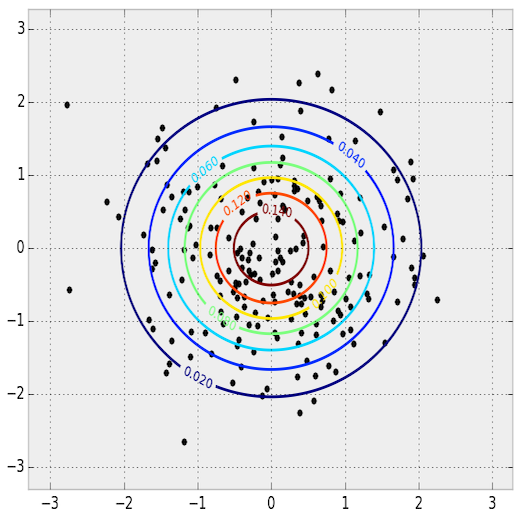## 第二步：快速生成伪造图像Yann LeCun 在这篇Quora 回答中介绍了对抗性训练。EyeScream 博文中的对抗训练的街头霸王游戏类比（Street Fighter analogy for adversarial networks）。（博文地址：http://soumith.ch/eyescream/）这些网络互相争斗并且一起发展进步，就像两个玩对战游戏的人类。

```z = np.random.uniform(-1, 1, 5)
array([ 0.77356483,  0.95258473, -0.18345086,  0.69224724, -0.34718733])```

```def G(z):
...
return imageSample
z = np.random.uniform(-1, 1, 5)
imageSample = G(z)```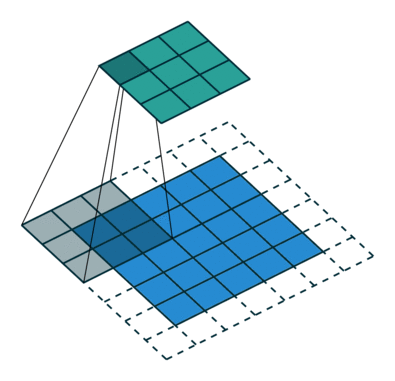一个从输入（蓝色）到输出（绿色）的卷积示意图。图像来源： vdumoulin/conv_arithmeticDCGAN 论文还展现了其他的技巧，还有对 DCGAN 训练的调节方法，比如用批归一化（batch normalization）或 leaky ReLU。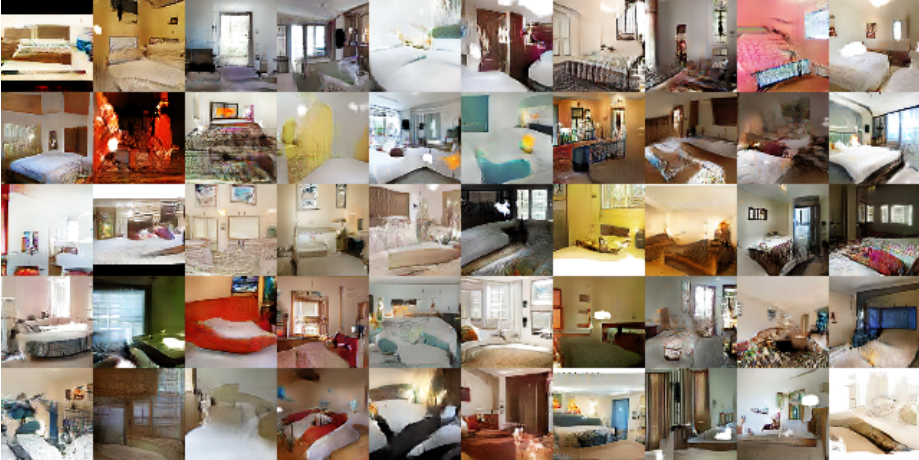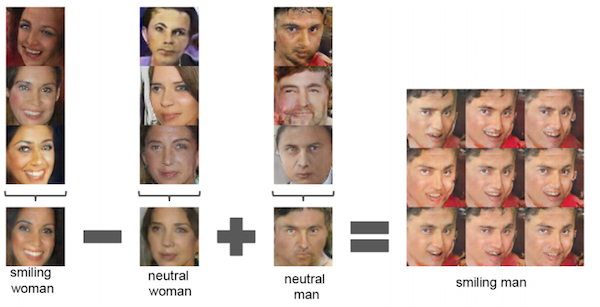[ML-Heavy]训练DCGANs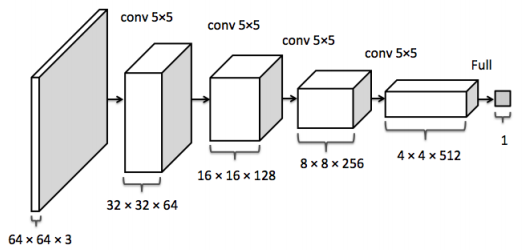1. 对于真实数据分布 x~ pdata中的每个图像最大化 D(x)。

2. 对于来自非真实数据分布 x≁ pdata 的每个图像把 D(x) 最小化。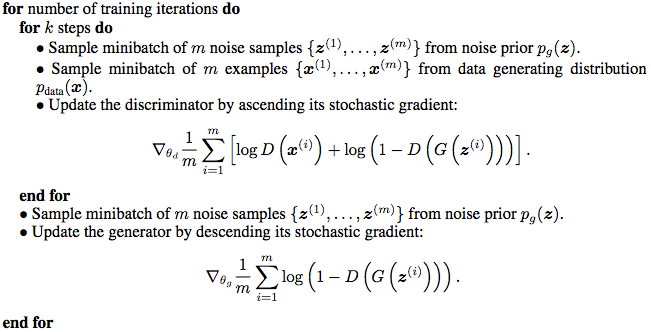GAN paper里的GAN训练算法

GitHub 上有很多好的 GAN 和 DCGAN 实现方法：

• goodfeli/adversarial (https://github.com/goodfeli/adversarial): GAN 论文作者放出的 Theano GAN 实现方法。 tqchen/mxnet-gan (https://github.com/tqchen/mxnet-gan): 非正式MXNet GAN实现。

• Newmu/dcgan_code (https://github.com/Newmu/dcgan_code): DCGAN 论文作者放出的Theano DCGAN 实现方法。

• soumith/dcgan.torch (https://github.com/soumith/dcgan.torch): DCGAN 论文作者之一（Soumith Chintala）放出的Torch DCGAN 实现方法。

• carpedm20/DCGAN-tensorflow (https://github.com/carpedm20/DCGAN-tensorflow): 非正式 TensorFlow DCGANU 实现。

• openai/improved-gan (https://github.com/openai/improved-gan):  OpenAI  (https://arxiv.org/abs/1606.03498)第一篇论文背后的代码。大幅修正了carpedm20/DCGAN-tensorflow。

• mattya/chainer-DCGAN (https://github.com/mattya/chainer-DCGAN):非正式 Chainer DCGAN 实现。

• jacobgil/keras-dcgan (https://github.com/jacobgil/keras-dcgan):  非正式（也不完整）Keras DCGAN 实现。

[ML-Heavy]TensorFlow上的 DCGANs

`self.d_loss_real = tf.reduce_mean(    tf.nn.sigmoid_cross_entropy_with_logits(self.D_logits,                                            tf.ones_like(self.D)))self.d_loss_fake = tf.reduce_mean(    tf.nn.sigmoid_cross_entropy_with_logits(self.D_logits_,                                            tf.zeros_like(self.D_)))self.d_loss = self.d_loss_real + self.d_loss_fakeself.g_loss = tf.reduce_mean(    tf.nn.sigmoid_cross_entropy_with_logits(self.D_logits_,                                            tf.ones_like(self.D_)))`

`t_vars = tf.trainable_variables()self.d_vars = [var for var in t_vars if 'd_' in var.name]self.g_vars = [var for var in t_vars if 'g_' in var.name]`

`d_optim = tf.train.AdamOptimizer(config.learning_rate, beta1=config.beta1) \                    .minimize(self.d_loss, var_list=self.d_vars)g_optim = tf.train.AdamOptimizer(config.learning_rate, beta1=config.beta1) \                    .minimize(self.g_loss, var_list=self.g_vars)`

```for epoch in xrange(config.epoch):
...
for idx in xrange(0, batch_idxs):
batch_images = ...
batch_z = np.random.uniform(-1, 1, [config.batch_size, self.z_dim]) \
.astype(np.float32)

# Update D network
＃更新一个 D 网络
_, summary_str = self.sess.run([d_optim, self.d_sum],
feed_dict={ self.images: batch_images, self.z: batch_z })

# Update G network
＃更新一个 G 网络
_, summary_str = self.sess.run([g_optim, self.g_sum],
feed_dict={ self.z: batch_z })

# Run g_optim twice to make sure that d_loss does not go to zero*
# (different from paper)
＃运行两次*g_optim 以确保 d_loss 不会变成0
＃（与论文里不一样）
_, summary_str = self.sess.run([g_optim, self.g_sum],
feed_dict={ self.z: batch_z })

errD_fake = self.d_loss_fake.eval({self.z: batch_z})
errD_real = self.d_loss_real.eval({self.images: batch_images})
errG = self.g_loss.eval({self.z: batch_z})```

```git clone https://github.com/cmusatyalab/openface.git
git clone https://github.com/bamos/dcgan-completion.tensorflow.git```

``` cd openface
pip2 install -r requirements.txt
python2 setup.py installcd ..```

`./openface/util/align-dlib.py data/dcgan-completion.tensorflow/data/your-dataset/raw align innerEyesAndBottomLip data/dcgan-completion.tensorflow/data/your-dataset/aligned --size 64`

`cd dcgan-completion.tensorflow/data/your-dataset/alignedfind . -name ' .png' -exec mv {} . \;find . -type d -empty -deletecd ../../..`

`./train-dcgan.py --dataset ./data/your-dataset/aligned --epoch 20`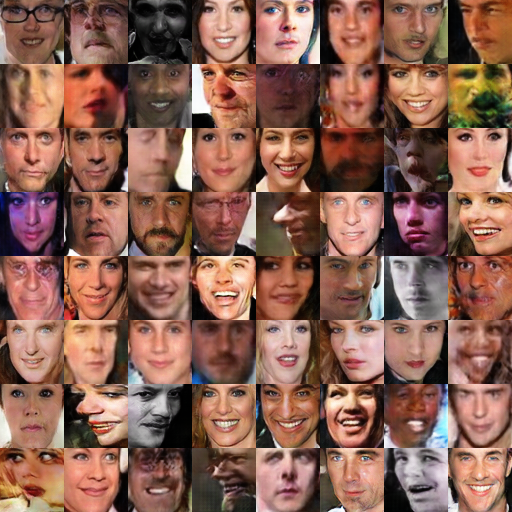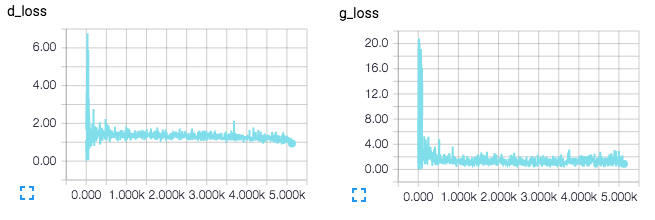TensorBoard 损耗的可视化，将在训练中实时更新。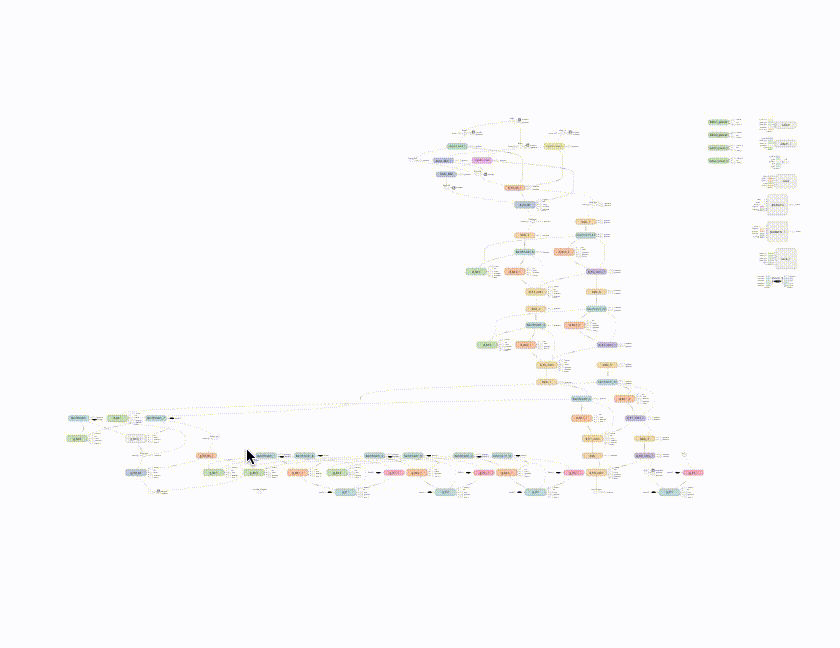DCGAN 网络的 TensorBoard 可视化。

## 第三步：找到修复图像的最佳伪造图片(a): y 在生成分布（蓝色）上的理想重建

(b): 试图只通过最大化 D(y) 重建 y 的失败例子。图像来源：inpainting paper

[ML-Heavy] pg上映射的损失函数||x||1=∑i|xi|是某些向量 x 的 ℓt 标准。  ℓ2 标准是另一个合理的选择，但那篇图像修复论文说  ℓ1 标准在实际应用中效果更好。在这个理想的情况下，所有已知位置的像素点在 y 和 G(z) 之间都是一样的。所以对已知像素点

G(z)i−yi= 0，因而Lcontextual(z)=0。λ 是一个控制输入环节缺失如何与直觉缺失相联系的超参数。（我用了默认的  λ=0.1，而且还没有太多改变它。）接着就跟前面一样，重构后的图像用 y （G(ẑ )）填补了缺失值：`self.mask = tf.placeholder(tf.float32, [None] + self.image_shape, name='mask')`

```self.contextual_loss = tf.reduce_sum(
tf.contrib.layers.flatten(
self.perceptual_loss = self.g_loss
self.complete_loss = self.contextual_loss + self.lam*self.perceptual_loss

``` if config.maskType == 'center':
scale = 0.25
assert(scale <= 0.5)
l = int(self.image_size*scale)
u = int(self.image_size*(1.0-scale))

``` for idx in xrange(0, batch_idxs):
batch_images = ...
zhats = np.random.uniform(-1, 1, size=(self.batch_size, self.z_dim))

v = 0
for i in xrange(config.nIter):
fd = {
self.z: zhats,
self.images: batch_images,
}
loss, g, G_imgs = self.sess.run(run, feed_dict=fd)

v_prev = np.copy(v)
v = config.momentum*v - config.lr*g
zhats += -config.momentum * v_prev + (1+config.momentum)*v
np.clip(zhats, -1, 1)
Completing your images```

`./complete.py ./data/your-test-data/aligned/***** --outDir outputImages`

`cd outputImagesconvert -delay 10 -loop 0 before.png completed/   .png completion.gif`## 结论

1. 把图像解析成一个概率分布中的样本点

2. 生成伪图像

3. 找到修复所需的最佳伪图像ImageNet 上的 DCGAN 样本（左）和改进后的 GAN 样本（右，本文中为涉及到），表明我们还没有理解如何对每种类型图像运用 GAN 。图像来源：improved GAN paper 。

### 扩展阅读